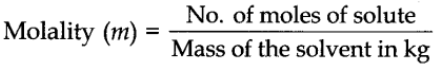• The word ‘stoichiometry’ is derived from two Greek words – Stoicheion (meaning element) and metron (meaning measure).
• The study of chemical reactions and calculations related to it is called Stoichiometry.
• The coefficients used to balance the reaction are called Stoichiometric Coefficients.
• The quantitative study of the reactants required or the products formed is called stoichiometry.
• Using stoichiometric calculations, the amounts of one or more reactant(s) required to produce a particular amount of product can be determined and vice-versa.

Let us now discuss the various conversion factors to solve stoichiometric problems. We have to follow a few steps for the same. They are:

• Balancing the given equation.
• Giving the substance the unit as a mole.
• Calculating the number of moles.

# Stoichiometric Calculations are Based on Chemical Formulas

Let’s learn some terms used in Stoichiometry first.

## Formula Mass:

It is the sum of the atomic weights of the various atoms present in the molecule of the substance. For example, we can calculate the formula mass of Na2S as 2(23) + 1(32) = 78

Avogadro’s number is the total number of particles present in one mole of a substance. It is the number of atoms present in exactly 12g of C-12. Avogadro number is valued as 6.022 × 1023.

## Molar Mass:

It is the sum of the total mass of all the atoms that constitute a molecule per mole.

Let us consider the combustion of methane. A balanced equation for this reaction is as given below: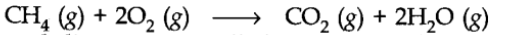Here, methane and dioxygen are called reactants and carbon dioxide and water are called products. (g) indicates that all the reactants and products are gases. The coefficients 2 for O2 and H2O are called stoichiometric coefficients. Thus, according to the above chemical reaction,From these relationships, the given data can be interconverted as follows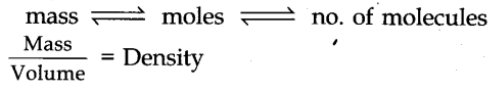# Limiting Reactant/ Reagent

Limiting reagent is an important concept in a chemical calculation. It refers to a reactant which is present in minimum stoichiometry quantity for a chemical reaction. This particular reactant is consumed fully in a chemical reaction. So, we make all the calculations related to various products or in a sequence of reactions on the basis of limiting reagent.

This reagent enters the picture when the reaction involves two or more reactants. For solving any such reactions, the first step is to calculate the limiting reagent.

# Reaction in Solutions

When the reactions are carried out in solutions, the amount of substance present in its given volume can be expressed in any of the following ways:

• Mass percent or weight percent (w/w%)
• Mole fraction
• Molarity
• Molality

# Mass percent or weight percent

It is obtained by using the following relation: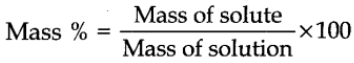## Mole fraction

It is the ratio of the number of moles of a particular component to the total number of moles of the solution. If a substance ‘A’ dissolves in substance ‘B’ and their number of moles are nA and nB respectively; then the mole fraction of A and B are given as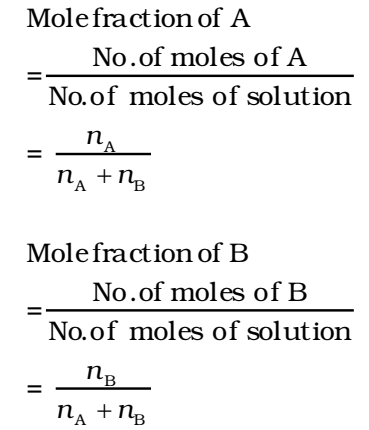The sum of the mole fractions of the components is equal to 1.

i.e., mole fraction of A + mole fraction of B = 1

## Molarity

It is defined as the number of moles of solute in 1 litre of the solution. It is denoted by the symbol M.In terms of weight, the molarity of the substance can be expressed as:## Molality

It is defined as the number of moles of solute present in 1 kg of solvent. It is denoted by m. It is denoted by the symbol m.